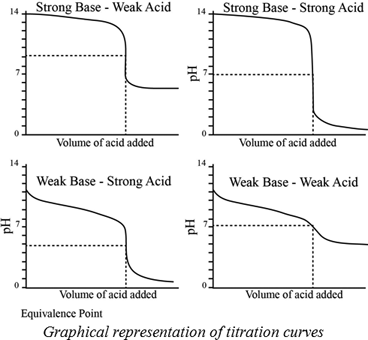Chapter 17, Problem 36PS

Chapter
Section
Textbook Problem

Using Figure 17.11, suggest an indicator to use in each of the following titrations. (a) NaHCO3 is titrated to CO32− with NaOH. (b) Hypochlorous acid is titrated with NaOH. (c) Trimethylamine is titrated with HCl.

a)

Interpretation Introduction

Interpretation:

NaHCO3 is titrated to CO32- with NaOH; an indicator used has to be suggested.

Concept introduction:

A titration is one of the most useful ways of determining accurately the quantity of an acid, a base or some other substances in a mixture.

The pH at the equivalence point of a strong acid –strong base titration is 7.

A weak acid titrated with a strong base leads to a pH > 7 at the equivalence point.

A weak base titrated with a strong acid leads to pH < 7 at the equivalence point.Indicators:

A chemical substances which gives a visible change  in the titration.

Some important indicators are as follow.Explanation

HCO3- is a weak acid and pKa= 10.32. When a weak acid is titrated with a strong base the pH at the equivalence point is above 7.

HCO3- have high pKa value therefore, it is weak base

b)

Interpretation Introduction

Interpretation:

Hypochlorous acid titrated with NaOH; an indicator used has to be suggested.

Concept introduction:

A titration is one of the most useful ways of determining accurately the quantity of an acid, a base or some other substances in a mixture.

The pH at the equivalence point of a strong acid –strong base titration is 7.

A weak acid titrated with a strong base leads to a pH > 7 at the equivalence point.

A weak base titrated with a strong acid leads to pH < 7 at the equivalence point.Indicators:

A chemical substances which gives a visible change  in the titration.

Some important indicators are as follow.c)

Interpretation Introduction

Interpretation:

Trimethylamine is titrated with HCl ; an indicator used has to be suggested.

Concept introduction:

A titration is one of the most useful ways of determining accurately the quantity of an acid, a base or some other substances in a mixture.

The pH at the equivalence point of a strong acid –strong base titration is 7.

A weak acid titrated with a strong base leads to a pH > 7 at the equivalence point.

A weak base titrated with a strong acid leads to pH < 7 at the equivalence point.Indicators:

A chemical substances which gives a visible change  in the titration.

Some important indicators are as follow.Still sussing out bartleby?

Check out a sample textbook solution.

See a sample solution

The Solution to Your Study Problems

Bartleby provides explanations to thousands of textbook problems written by our experts, many with advanced degrees!

Get Started